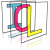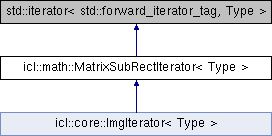Image Component Library (ICL)
icl::math::MatrixSubRectIterator< Type > Class Template Reference

Iterator class used to iterate through a sub rect of 2D data. More...

`#include <MatrixSubRectIterator.h>`

Inheritance diagram for icl::math::MatrixSubRectIterator< Type >:## Public Member Functions

MatrixSubRectIterator ()
Default Constructor. More...

MatrixSubRectIterator (Type *ptData, int matrixWidth, int subRectX, int subRectY, int subRectWidth, int subRectHeight)

MatrixSubRectIteratorassign (const MatrixSubRectIterator &other)

const MatrixSubRectIteratoroperator= (const MatrixSubRectIterator &other) const

const Type & operator * () const
retuns a reference of the current pixel value (const) More...

Type & operator * ()
retuns a reference of the current pixel value More...

MatrixSubRectIteratoroperator++ ()
moves to the next iterator position (Prefix ++it) More...

const MatrixSubRectIteratoroperator++ () const
const version of pre increment operator More...

MatrixSubRectIterator operator++ (int)

const MatrixSubRectIterator operator++ (int) const
const version of post increment operator More...

bool inSubRect () const
to check if iterator is still inside the ROI More...

bool operator!= (const MatrixSubRectIterator< Type > &it) const
compare two iterators More...

bool operator== (const MatrixSubRectIterator< Type > &it) const
compare two iterators More...

bool operator< (const MatrixSubRectIterator< Type > &it) const
compare two iterators More...

bool operator> (const MatrixSubRectIterator< Type > &it) const
compare two iterators More...

bool operator<= (const MatrixSubRectIterator< Type > &it) const
compare two iterators More...

bool operator>= (const MatrixSubRectIterator< Type > &it) const
compare two iterators More...

int getSubRectWidth () const
returns the length of each row processed by this iterator More...

int getSubRectHeight () const

void incRow (int numLines=1) const
move the pixel vertically forward More...

int x ()
returns the current x position of the iterator (wrt matrix origin); More...

int y ()
returns the current y position of the iterator (wrt matrix origin) More...

## Static Public Member Functions

static const MatrixSubRectIterator< Type > create_end_iterator (const Type *dataOrigin, int matrixWidth, int subRectX, int subRectY, int subRectWidth, int subRectHeight)

void init ()

## Protected Attributes

int m_matrixWidth
corresponding matrix width More...

int m_subRectWidth
sub rect size of the iterator More...

int m_subRectHeight

int m_lineStep
result of m_matrixWidth - m_subRectWidth More...

Type * m_dataOrigin
pointer to the upper matrix data origin (upper left element) More...

Type * m_dataCurr
pointer to the current data element More...

Type * m_dataEnd
pointer to the first element behind the subrect More...

Type * m_currLineEnd
pointer to the first invalid element of the current line More...

## Detailed Description

### template<typename Type> class icl::math::MatrixSubRectIterator< Type >

Iterator class used to iterate through a sub rect of 2D data.

```The MatrixSubRectIterator is a utility to iterate line by line through
all elements of a sub-rectangle of a row-major-order aligned data block.
```

The following ASCII image shows an images ROI.

```  1st element
|
....|....................
....+->Xoooooooo......... ---
.......ooooooooo.........  |
.......ooooooooo......... Rect-Height
.......ooooooooo.........  |
.......ooooooooo......... ---
.........................
|-RectW-|
|---------MatrixW-------|```
```\section CONST const-ness
Please note that the const-ness of an MatrixSubRectIterator instance does
not say anything about the sturcture itselft. Hence also const
MatrixSubRectIterators can be 'moved' using ++-operators or incRow()
method.\n
Instead, const-ness relates to the underlying data block
is referenced by the iterator instance.
```

## ◆ MatrixSubRectIterator() [1/2]

template<typename Type>
 icl::math::MatrixSubRectIterator< Type >::MatrixSubRectIterator ( )
inline

Default Constructor.

Creates an MatrixSubRectIterator object

## ◆ MatrixSubRectIterator() [2/2]

template<typename Type>
 icl::math::MatrixSubRectIterator< Type >::MatrixSubRectIterator ( Type * ptData, int matrixWidth, int subRectX, int subRectY, int subRectWidth, int subRectHeight )
inline

2nd Constructor creates an MatrixSubRectIterator object with Type "Type"

Parameters
 ptData pointer to the corresponding channel data matrixWidth width of the corresponding image subRectX ROI upper-left X-coordinate subRectY ROI upper-left Y-coordinate subRectWidth ROI width subRectHeight ROI height

## ◆ assign()

template<typename Type>
 MatrixSubRectIterator& icl::math::MatrixSubRectIterator< Type >::assign ( const MatrixSubRectIterator< Type > & other )
inline

## ◆ create_end_iterator()

template<typename Type>
 static const MatrixSubRectIterator icl::math::MatrixSubRectIterator< Type >::create_end_iterator ( const Type * dataOrigin, int matrixWidth, int subRectX, int subRectY, int subRectWidth, int subRectHeight )
inlinestatic

## ◆ getSubRectHeight()

template<typename Type>
 int icl::math::MatrixSubRectIterator< Type >::getSubRectHeight ( ) const
inline

## ◆ getSubRectWidth()

template<typename Type>
 int icl::math::MatrixSubRectIterator< Type >::getSubRectWidth ( ) const
inline

returns the length of each row processed by this iterator

Returns
row length

## ◆ incRow()

template<typename Type>
 void icl::math::MatrixSubRectIterator< Type >::incRow ( int numLines = `1` ) const
inline

move the pixel vertically forward

current x value is hold, the current y-value is incremented by iLines

Parameters
 numLines amount of lines to jump over

## ◆ init()

template<typename Type>
 void icl::math::MatrixSubRectIterator< Type >::init ( )
inlineprotected

## ◆ inSubRect()

template<typename Type>
 bool icl::math::MatrixSubRectIterator< Type >::inSubRect ( ) const
inline

to check if iterator is still inside the ROI

This function was replaced by STL-like begin(), end() logic Although in some cases it might be quite useful, so we renamed it rather than deleting it

operator++

## ◆ operator *() [1/2]

template<typename Type>
 const Type& icl::math::MatrixSubRectIterator< Type >::operator * ( ) const
inline

retuns a reference of the current pixel value (const)

changes on *p (p is of type MatrixSubRectIterator) will effect the image data

## ◆ operator *() [2/2]

template<typename Type>
 Type& icl::math::MatrixSubRectIterator< Type >::operator * ( )
inline

retuns a reference of the current pixel value

changes on *p (p is of type MatrixSubRectIterator) will effect the image data

## ◆ operator!=()

template<typename Type>
 bool icl::math::MatrixSubRectIterator< Type >::operator!= ( const MatrixSubRectIterator< Type > & it ) const
inline

compare two iterators

## ◆ operator++() [1/4]

template<typename Type>
 MatrixSubRectIterator& icl::math::MatrixSubRectIterator< Type >::operator++ ( )
inline

moves to the next iterator position (Prefix ++it)

The image ROI will be scanned line by line beginning on the bottom left iterator.

```       +-- begin here (index 0)
|
.......|.................
.......V.................
.......012+-->+8<---------- first line wrap after
.......9++++++++.........   this pixel (index 8)
.......+++++++++.........
.......+++++++++.........
.......++++++++X<---------- last valid pixel
....+->I.................
|
'I' is the first invalid iterator
(p.inRegion() will become false)```
`   `

In most cases The ++ operator will just increase the current x position and update the reference to the current pixel data. If the end of a line is reached, then the position is set to the beginning of the next line.

## ◆ operator++() [2/4]

template<typename Type>
 const MatrixSubRectIterator& icl::math::MatrixSubRectIterator< Type >::operator++ ( ) const
inline

const version of pre increment operator

## ◆ operator++() [3/4]

template<typename Type>
 MatrixSubRectIterator icl::math::MatrixSubRectIterator< Type >::operator++ ( int )
inline

postfix operator++ (used -O3 to avoid loss of performace when using the "it++"-operator In most cases the "++it"-operator will ensure best performace.

## ◆ operator++() [4/4]

template<typename Type>
 const MatrixSubRectIterator icl::math::MatrixSubRectIterator< Type >::operator++ ( int ) const
inline

const version of post increment operator

## ◆ operator<()

template<typename Type>
 bool icl::math::MatrixSubRectIterator< Type >::operator< ( const MatrixSubRectIterator< Type > & it ) const
inline

compare two iterators

## ◆ operator<=()

template<typename Type>
 bool icl::math::MatrixSubRectIterator< Type >::operator<= ( const MatrixSubRectIterator< Type > & it ) const
inline

compare two iterators

## ◆ operator=()

template<typename Type>
 const MatrixSubRectIterator& icl::math::MatrixSubRectIterator< Type >::operator= ( const MatrixSubRectIterator< Type > & other ) const
inline

## ◆ operator==()

template<typename Type>
 bool icl::math::MatrixSubRectIterator< Type >::operator== ( const MatrixSubRectIterator< Type > & it ) const
inline

compare two iterators

## ◆ operator>()

template<typename Type>
 bool icl::math::MatrixSubRectIterator< Type >::operator> ( const MatrixSubRectIterator< Type > & it ) const
inline

compare two iterators

## ◆ operator>=()

template<typename Type>
 bool icl::math::MatrixSubRectIterator< Type >::operator>= ( const MatrixSubRectIterator< Type > & it ) const
inline

compare two iterators

## ◆ x()

template<typename Type>
 int icl::math::MatrixSubRectIterator< Type >::x ( )
inline

returns the current x position of the iterator (wrt matrix origin);

Returns
current x position

## ◆ y()

template<typename Type>
 int icl::math::MatrixSubRectIterator< Type >::y ( )
inline

returns the current y position of the iterator (wrt matrix origin)

Returns
current y position

## ◆ m_currLineEnd

template<typename Type>
 Type* icl::math::MatrixSubRectIterator< Type >::m_currLineEnd
mutableprotected

pointer to the first invalid element of the current line

## ◆ m_dataCurr

template<typename Type>
 Type* icl::math::MatrixSubRectIterator< Type >::m_dataCurr
mutableprotected

pointer to the current data element

## ◆ m_dataEnd

template<typename Type>
 Type* icl::math::MatrixSubRectIterator< Type >::m_dataEnd
protected

pointer to the first element behind the subrect

## ◆ m_dataOrigin

template<typename Type>
 Type* icl::math::MatrixSubRectIterator< Type >::m_dataOrigin
protected

pointer to the upper matrix data origin (upper left element)

## ◆ m_lineStep

template<typename Type>
 int icl::math::MatrixSubRectIterator< Type >::m_lineStep
protected

result of m_matrixWidth - m_subRectWidth

## ◆ m_matrixWidth

template<typename Type>
 int icl::math::MatrixSubRectIterator< Type >::m_matrixWidth
protected

corresponding matrix width

## ◆ m_subRectHeight

template<typename Type>
 int icl::math::MatrixSubRectIterator< Type >::m_subRectHeight
protected

## ◆ m_subRectWidth

template<typename Type>
 int icl::math::MatrixSubRectIterator< Type >::m_subRectWidth
protected

sub rect size of the iterator

The documentation for this class was generated from the following file: